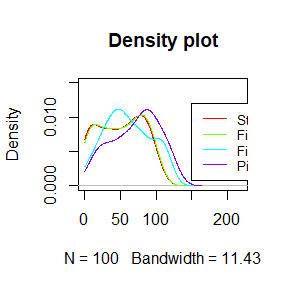# Introduction

The purpose of the package queuecomputer is to compute, deterministically, the output of a queue network given the arrival and service times for all customers. The most important functions are queue_step, lag_step and wait_step.

# Input format

The first argument to the functions queue_step, lag_step and wait_step is a vector of arrival times. For example:

library(queuecomputer)
library(dplyr)
##
## Vedhæfter pakke: 'dplyr'
## De følgende objekter er maskerede fra 'package:stats':
##
##     filter, lag
## De følgende objekter er maskerede fra 'package:base':
##
##     intersect, setdiff, setequal, union
arrivals <- cumsum(rexp(100))

head(arrivals)
##  1.177217 2.548002 2.633025 4.583521 6.300688 6.953988
service <- rexp(100)

departures <- queue_step(arrivals = arrivals, service = service)

str(departures,1)
## List of 7
##  $departures : num [1:100] 2.45 2.93 3.7 5.4 7.07 ... ##$ server         : int [1:100] 1 1 1 1 1 1 1 1 1 1 ...
##  $departures_df : tibble [100 x 6] (S3: tbl_df/tbl/data.frame) ##$ queuelength_df :'data.frame': 201 obs. of  2 variables:
##  $systemlength_df:'data.frame': 201 obs. of 2 variables: ##$ servers_input  : num 1
##  $state : num 101 ## - attr(*, "class")= chr [1:2] "queue_list" "list" # Resourcing schedule The resourcing schedule is specified with either a non-zero natural number, a server.stepfun or a server.list object. Use a non-zero natural number when the number of servers does not change over time. The server.stepfun specifies a step function to indicate how many servers are available throughout the day. The computation speed for queue_step() is much faster when using a server.stepfun rather than a server.list input for the servers argument. We create a server.stepfun object with the as.server.stepfun function. # Zero servers available before time 10 # One server available between time 10 and time 50 # Three servers available between time 50 and time 100 # One server available from time 100 onwards resource_schedule <- as.server.stepfun(c(10,50,100), c(0, 1, 3, 1)) resource_schedule ##$x
##   10  50 100
##
## $y ##  0 1 3 1 ## ## attr(,"class") ##  "server.stepfun" "list" departures <- queue_step(arrivals = arrivals, service = service, servers = resource_schedule) str(departures,1) ## List of 7 ##$ departures     : num [1:100] 11.3 11.7 12.4 13.2 14 ...
##  $server : int [1:100] 1 1 1 1 1 1 1 1 1 1 ... ##$ departures_df  : tibble [100 x 6] (S3: tbl_df/tbl/data.frame)
##  $queuelength_df :'data.frame': 201 obs. of 2 variables: ##$ systemlength_df:'data.frame': 201 obs. of  2 variables:
##  $servers_input :List of 2 ## ..- attr(*, "class")= chr [1:2] "server.stepfun" "list" ##$ state          : num [1:3] 93.8 91.6 90.8
##  - attr(*, "class")= chr [1:2] "queue_list" "list"

The server.list object is a list of step functions which represent each server, the range is $$\{0,1\}$$, where 0 represents unavailable and 1 represents available and the knots represent the times where availability changes.

The as.server.list function is used to create a server.list object.

# Server 1 is available before time 10.
# Server 2 is available between time 15 and time 30.
# Server 3 is available after time 10.
as.server.list(list(10, c(15,30), 10), c(1,0,0))
## []
## Step function
## Call: stats::stepfun(times[[i]], y)
##  x[1:1] =     10
## 2 plateau levels =      1,      0
##
## []
## Step function
## Call: stats::stepfun(times[[i]], y)
##  x[1:2] =     15,     30
## 3 plateau levels =      0,      1,      0
##
## []
## Step function
## Call: stats::stepfun(times[[i]], y)
##  x[1:1] =     10
## 2 plateau levels =      0,      1
##
## attr(,"class")
##  "list"        "server.list"

# Setting up a queue network

It is simple to set up a chain of queueing elements with queuecomputer. Suppose passengers must walk to a queue, then wait for service and then wait for their bags.

library(queuecomputer)
library(dplyr)

set.seed(500)

n <- 100

arrivals <- cumsum(rexp(n))
service_l <- rexp(n, 0.8)
service_q <- rexp(n, 0.5)
arrivals_b <- cumsum(rexp(n, 0.8))

# The queue elements can be computed one by one.

departures_1 <- lag_step(arrivals, service_l)
departures_2 <- queue(departures_1, service = service_q, servers = 2)
departures_3 <- wait_step(departures_2, arrivals_b)

# Or the queue elements can be chained together with the %>% operator.

departures <- lag_step(arrivals, service_l) %>% queue_step(service = service_q, servers = 2) %>% wait_step(arrivals_b)

all(departures == departures_3)
##  TRUE
# Plot densities for this tandem queueing network

colours <- rainbow(4)
plot(density(arrivals, from = 0),
col = colours, xlim = c(0, 220), ylim = c(0, 0.015),
main = "Density plot")
lines(density(departures_1, from = 0), col = colours)
lines(density(departures_2, from = 0), col = colours)
lines(density(departures_3, from = 0), col = colours)
legend(150,0.012, legend = c("Start walk",
"Finish walk",
"Finish service",
"Pick up bag"),
col = colours, lwd = 1, cex = 0.8
)